How Cheenta works to ensure student success?
Explore the Back-Story

# NMTC 2019 Stage II - BHASKARA (Class 9, 10) - Problems and Solutions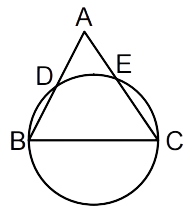###### Problem 1

In a convex quadrilateral PQRS, the areas of triangles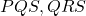andare in the ratio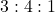. A line throughcuts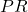atandat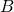such that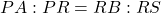. Prove thatis the midpoint ofandis the midpoint of.

###### Problem 2

Given positive real numbers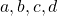such that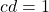. Prove that there exist at least one positive integer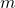such that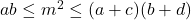.

###### Problem 3

Find the number of permutations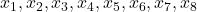of the integers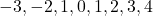that satisfy the chain of inequalities.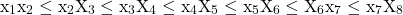###### Problem 4

In the figure,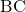is a diameter of the circle, where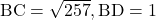, and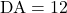. Find the length ofand hence find the length of the altitude from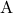to.

###### Problem 5

A math contest consists of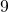objective type questions and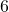fill in the blanks questions. From a school some number of students took the test and it was noticed that all students had attempted exactly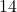out of the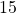questions. Let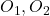,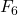be the six fill in the blanks questions. Let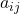be the number of students who attempted both questions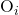and. If the sum of all the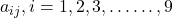and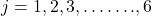is, then find the number of students who took the test in the school.

###### Problem 6

Find all positive integer triples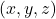that satisfy the equation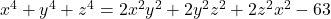###### Problem 7

The perimeter ofis 2 and its sides are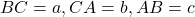. Prove that.

###### Problem 8

A circular disc is divided into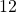equal sectors and one ofdifferent colours is used to colour each sector. No two adjacent sectors can have the same colour. Find the number of such distinct colourings possible.

###### Problem 1

In a convex quadrilateral PQRS, the areas of trianglesandare in the ratio. A line throughcutsatandatsuch that. Prove thatis the midpoint ofandis the midpoint of.

###### Problem 2

Given positive real numberssuch that. Prove that there exist at least one positive integersuch that.

###### Problem 3

Find the number of permutationsof the integersthat satisfy the chain of inequalities.###### Problem 4

In the figure,is a diameter of the circle, where, and. Find the length ofand hence find the length of the altitude fromto.

###### Problem 5

A math contest consists ofobjective type questions andfill in the blanks questions. From a school some number of students took the test and it was noticed that all students had attempted exactlyout of thequestions. Let,be the six fill in the blanks questions. Letbe the number of students who attempted both questionsand. If the sum of all theandis, then find the number of students who took the test in the school.

###### Problem 6

Find all positive integer triplesthat satisfy the equation###### Problem 7

The perimeter ofis 2 and its sides are. Prove that.

###### Problem 8

A circular disc is divided intoequal sectors and one ofdifferent colours is used to colour each sector. No two adjacent sectors can have the same colour. Find the number of such distinct colourings possible.

This site uses Akismet to reduce spam. Learn how your comment data is processed.

### Knowledge Partner